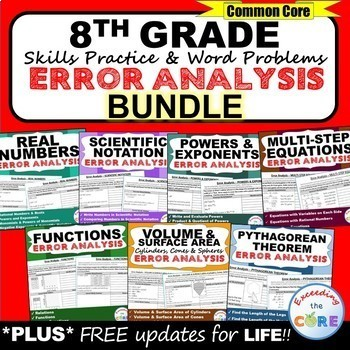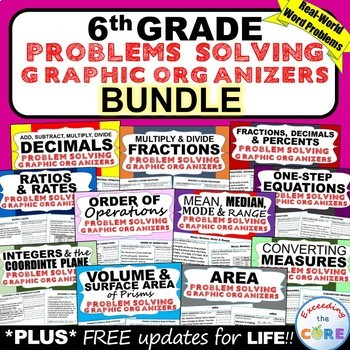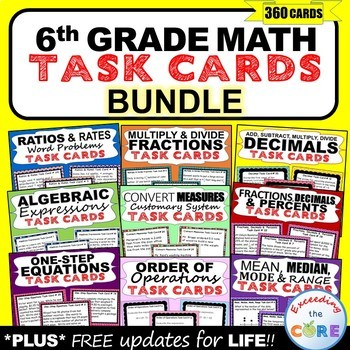### BUNDLE UP and save BIG with my MATH BUNDLES

I am so happy to highlight these amazing Middle School MATH BUNDLES!!  They are full of great resources that I know you and your students will find very useful in your math classroom.  Each one includes real-world word problems that will engage your students and push their mathematical thinking.

{{CLICK ON THE IMAGES to learn more about each resources.}}

MATH ERROR ANALYSIS
Each page includes a real-world word problems that is solved incorrectly. Students have to identify the error, provide the correct solution and share a helpful strategy for solving the problem.6th Grade Math Error Analysis
7th Grade Math Error Analysis
8th Grade Math Error Analysis

MATH PROBLEM SOLVING GRAPHIC ORGANIZERS

Each worksheet presents students with a real-word problem. Students must then organize the information using a problem solving graphic organizer. Students are prompted to identify the important information in the word problem, solve, justify their work and explain their solution.6th Grade Math Problem Solving Graphic Organizers
7th Grade Math Problem Solving Graphic Organizers
8th Grade Math Problem Solving Graphic Organizers